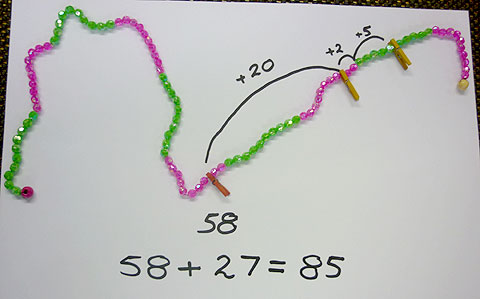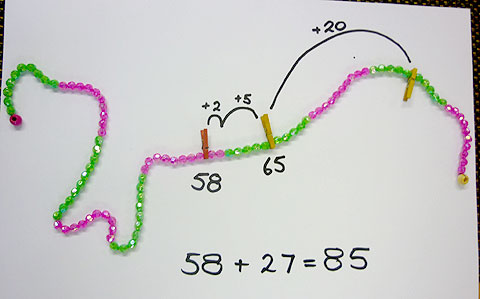# Renaming to add and subtract

Pose addition and subtraction problems -- in context -- that can be solved using place value with renaming.

In addition problems this involves combining ones to form a ten.

For example, 57 + 28 = ? requires combining 7 and 8 to form 10 and 5.

You can watch the Renaming Tens with Addition video.

You can download the Renaming Tens with Addition video transcript.

In subtraction we decompose tens when more ones need to be taken away than are immediately available.

For example, 83 – 47 = ? requires subtraction of seven ones when only three are immediately available.

You can watch the Renaming Tens with Subtraction video.

You can download the Renaming Tens with Subtraction video transcript.

Both of these videos illustrate renaming strategies based on splitting tens. Renaming problems can also be solved using jumping strategies. Jumping tends to be more reliable as a mental strategy because it removes the memory load associated with the starting number.Adding with a bead string (solution 1).Adding with a bead string (solution 2).

Jumping strategies can be practised using the empty number line applet on the New Zealand Maths website.

These ideas are explored further in Number Facts: Addition and Subtraction and Fluency and Flexibility.